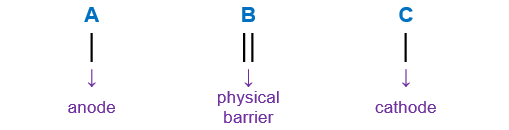# Problem: For the following electrochemical cell        Co(s)|Co2+(aq, 0.0155 M)||Ag+(aq, 1.50 M)|Ag(s)write the net cell equation. Phases are optional. Do not include the concentrations.Calculate the following values at 25.0 °C using standard potentials as needed.E°cell =            V                  ΔG°rxn =           kJ/molEcell =            V                  ΔGrxn =           kJ/mol

###### FREE Expert Solution

Part 1: To write the overall balanced or net cell equation for the given cell notation:

Co(s)|Co2+(aq, 0.0155 M)||Ag+(aq, 1.50 M)|Ag(s)

When writing a cell notation, we use the following format – “as easy as ABCRecall the mnemonics LEO GER.

79% (215 ratings)###### Problem Details

For the following electrochemical cell

Co(s)|Co2+(aq, 0.0155 M)||Ag+(aq, 1.50 M)|Ag(s)

write the net cell equation. Phases are optional. Do not include the concentrations.

Calculate the following values at 25.0 °C using standard potentials as needed.

cell =            V                  ΔG°rxn =           kJ/mol

Ecell =            V                  ΔGrxn =           kJ/mol